# 2.6.2.7.4 Emulators

Page 38 of 54

The purpose of a statistical emulator is to provide a computationally efficient surrogate for a computationally expensive model. These emulators provide a way to quantify the predictive distribution for a prediction of interest, given a new set of parameters at which the model was not run. As outlined in the uncertainty analysis workflow (Figure 6 in Section 2.6.2.1), an emulator is created for each objective function (see Section 2.6.2.8.1) and for each prediction of additional drawdown and year of maximum change. The objective function emulators are used in the Markov chain Monte Carlo sampling of the prior parameter distribution to create a posterior parameter distribution for each prediction. These posterior parameter distributions are subsequently sampled with the emulator for that prediction to produce the predictive distribution of additional drawdown and year of maximum change at each model node.

The statistical emulation approach employed herein is called Local Approximate Gaussian Processes (LAGPs) as implemented through the ‘aGP’ function of the ‘laGP’ package (Gramacy, 2014) for R (R Core Team, 2013). LAGPs were chosen because: (i) they can be built and run very rapidly in the ‘laGP’ R package; (ii) unlike some other popular emulation approaches (e.g. standard Gaussian process emulators), they allow for nonstationarity in the model output across the parameter space, which provides the emulator with more flexibility to match model output; and (iii) they were found to have excellent performance when compared to a range of other emulation techniques (Nguyen-Tuong et al., 2009; Gramacy, 2014).

The training and evaluating of an individual emulator is implemented through a set of custom-made R-scripts with following input requirements:

• design of experiment parameter combinations
• design of experiment model output
• transform of parameters
• transform of output.

Among the 3877 successfully finished model runs, 121 models were filtered out because their modeling results were considered unrealistic. The set of 3756 parameter combinations that were evaluated and their corresponding model results are used to train the individual emulators for each prediction. As noted before, predictions of additional drawdown less than the convergence head criterion of 0.001 m are considered numerical noise. These values are replaced with zero and the associated year of maximum change values are replaced with 2102, the end of the simulation period. In other words, any predicted drawdown of less than 0.001 m is considered to be equal to no drawdown within the simulation period.

The model parameters were transformed according to the transformations listed in Table 8. Before training the emulator, the quantity to be emulated is transformed using a normal quantile transform (Bogner et al., 2012). The following steps are required to carry out such a normal quantile transformation of a sample X:

1. Sort the sample X from smallest to largest: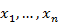2. Estimate the cumulative probabilities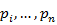using a plotting position like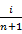such that3. Transform each valueof X in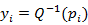of the normal variate Y, where Q-1 is the inverse of the standard normal distribution, using a discrete mapping.

The main advantage of this transformation is that it transforms any arbitrary distribution of values into a normal distribution. Gaussian process emulators tend to perform better if the quantity to emulate is close to the normal distribution. The drawback of the transformation is that it cannot be reliably used to extrapolate beyond the extremes of the distribution. This risk is minimised in this application by purposely choosing the parameter ranges in the design of experiment to be very wide as to encompass the plausible parameter range. In a final step, the resulting value of the emulator is back-transformed to the original distribution.

The predictive capability of LAGP emulators is assessed via 30-fold cross validation (i.e. leaving out 1/30th of the model runs, over 30 tests) and recording diagnostic plots of the emulator’s predictive capacity. For each of the 30 runs of the cross-validation procedure, the proportion of 95% predictive distributions that contained the actual values output by the model (also called the hit rate) was recorded. The emulators are considered sufficiently accurate if the 95% hit rate is between 90 and 100%.Figure 33 Scatterplots of the simulated average surface water - groundwater flux (SW-GW flux) between 1983 to 2012 at the Casino surface water model node (CLM_008) versus the parameter values of the evaluated design of experiment model runs

The accuracy of the emulator, the degree to which the emulator can reproduce the relationship between the parameters and the prediction, depends greatly on the density of sampling of parameter space. This section examines whether the set of 3756 evaluated parameter combinations provides sufficient information to train emulators. As it would be beyond the scope of this report to examine this for all of the emulators created, the suitability of the number of parameter combinations is illustrated using the average predicted surface watergroundwater flux in September and October at the Casino surface water model node (CLM_008). Emulating this quantity is especially challenging as the flux varies over at least two orders of magnitude and the flux is a non-linear function of several interacting parameters (Figure 33).Figure 34 Scatterplots of modelled versus emulated values of average surface water - groundwater flux at Casino between 1983 and 2012 for emulators trained with different training set sizes

Figure 34 (plots a to d) shows the correspondence between modelled and emulated flux predictions using an emulator trained with 1000, 2000, 3000, and 3700 samples, respectively. Emulators trained with less than 2000 samples do not perform well in emulating the flux. The correspondence between modelled and emulated values for the training set of 3700 samples is very high however, with the largest residuals at the high extreme of the flux distribution.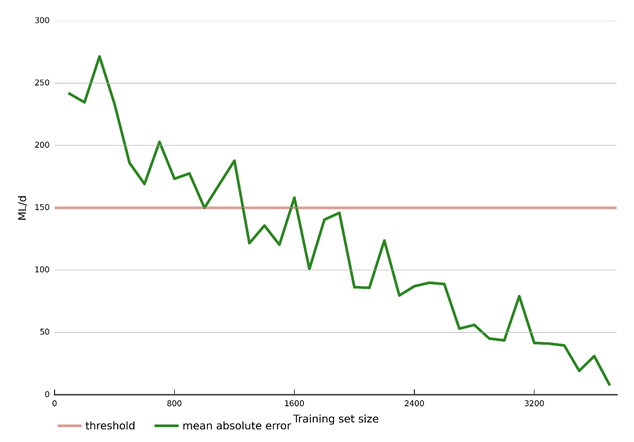Figure 35 Convergence of mean absolute error between modelled and emulated average surface water - groundwater flux at Casino between 1983 and 2012

Figure 35 shows this evolution in a more quantitative way by visualising the mean absolute error between modelled and emulated flux values produced by emulators trained with training sets that vary from 100 to 3756 in increments of 100. In the uncertainty analysis (Section 2.6.2.8.1), a parameter combination that results in a flux of less than 150 ML/day is accepted. The mean absolute error drops below that threshold value for emulators trained with more than 1600 samples. The emulator trained with the full training set has a mean absolute error of 8.2 ML/day. By using an emulator with this accuracy in the Markov chain Monte Carlo sampling, the risk of wrongly accepting or rejecting a parameter combination is very small. Emulators with this level of accuracy also provide confidence that predictions obtained with the emulators are very close to predictions generated with the original model.

Last updated:
7 January 2019